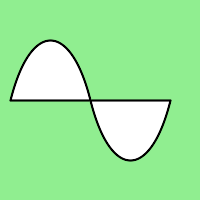Related Articles

# Wand path_close() function in Python

• Last Updated : 02 Jun, 2021

path_move() is another function included in wand for paths. The main aim of this function is to join the last destination point to the first point in the path. It simply adds a path element to the current path which closes the current subpath by drawing a straight line from the current point to the current subpath’s most recent starting point.

Syntax : draw.path_close()
Parameters : No parameters in this function.

Code :

## Python3

 `# importing wand``from` `wand.image ``import` `Image``from` `wand.drawing ``import` `Drawing``from` `wand.color ``import` `Color` `with Drawing() as draw:``    ``draw.stroke_width ``=` `2``    ``draw.stroke_color ``=` `Color(``'black'``)``    ``draw.fill_color ``=` `Color(``'white'``)``    ``draw.path_start()``    ``# Start middle-left``    ``draw.path_move(to ``=``(``10``, ``100``))``    ``# Curve across top-left to center``    ``draw.path_curve(to ``=``(``80``, ``0``),``                    ``controls ``=``[(``20``, ``-``80``), (``60``, ``-``80``)],``                    ``relative ``=` `True``)``    ``# Continue curve across bottom-right``    ``draw.path_curve(to ``=``(``80``, ``0``),``                    ``controls ``=``(``60``, ``80``),``                    ``smooth ``=` `True``,``                    ``relative ``=` `True``)``    ``# Join the last destination point to the first point``    ``draw.path_close()``    ``draw.path_finish()``    ``with Image(width ``=` `200``, height ``=` `200``, background ``=` `Color(``'lightgreen'``)) as image:``        ``draw(image)``        ``image.save(filename ``=` `"pathclose.png"``)`

Output:Attention geek! Strengthen your foundations with the Python Programming Foundation Course and learn the basics.

To begin with, your interview preparations Enhance your Data Structures concepts with the Python DS Course. And to begin with your Machine Learning Journey, join the Machine Learning – Basic Level Course

My Personal Notes arrow_drop_up